# Term Project

Part 1. Univariate Statistics.

The data below was obtained from Professor Kenneth French’s data library website:

Don't use plagiarized sources. Get Your Custom Essay on
Term Project
Get a plagiarism free paperJust from \$13/Page

http://mba.tuck.dartmouth.edu/pages/faculty/ken.french/data_library.html

The table below contains monthly returns of the “Fama/French 5 Factors” and the monthly returns of the “Momentum factor” for the period from July 1963-December 2017 (654 months)

• RM-RF The return spread between the capitalization weighted stock market and cash.
• SMB The return spread of small minus large stocks (i.e., the size effect).
• HML The return spread of cheap minus expensive stocks (i.e., the value effect).
• RMW The return spread of the most profitable firms minus the least profitable.
• CMA The return spread of firms that invest conservatively minus aggressively.
• MOM The retun spread of firms with high prior return minus low prior return.

1. Split the sample in 3 equal periods and compute the average, SD, skew, and kurtosis for each of the six “risk factors” for the full sample and the three different periods. Arrange these values in a table similar to the one shown below (5p).
 Full Sample: 1963M07 – 2017M12 MKT_RF SMB HML RMW CMA MOM Mean Std. Dev. Skewness Kurtosis Observations First sub-sample: 1963M07 – 1981M08 MKT_RF SMB HML RMW CMA MOM Mean Std. Dev. Skewness Kurtosis Observations Second sub-sample: 1981M09 – 1999M10 MKT_RF SMB HML RMW CMA MOM Mean Std. Dev. Skewness Kurtosis Observations Third sub-sample: 1999M11 – 2017M12 MKT_RF SMB HML RMW CMA MOM Mean Std. Dev. Skewness Kurtosis Observations

1. Do the statistics suggest to you that returns for those risk factors come from the same distribution over the entire period? (5p)

1. Make a plot showing the growth of \$1 in each of the six “risk factors (portfolios)” over the full sample. (Recall, this is called an “equity curve”). (5p)

1. Which factor portfolio gives the lowest and highest future value (full sample)? (5P)

1. Make a plot showing the growth of \$1 in each of the six “risk factors (portfolios)” over the five year period (Jan 2013 – Dec 2017). (Recall, this is called an “equity curve”). (5p)

1. Which factor portfolio gives the lowest and highest future value? (sample: last five years) (5p)

1. Give a brief explanation of what are the real, macroeconomic, aggregate, nondiversifiable risk that are proxied by the returns of the [RM-RF], SMB, HML, RMW, CMA and MOM risk portfolios. For example, why are investors so concerned about holding stocks that do badly at the times that the HML (value less growth) and SMB (small-cap less large-cap) portfolios do badly, even though the market [RM-RF] does not fall? (5p)

Part 2.

Go to Yahoo! finance site. Please download monthly adj. close prices from 12/1/2012 to 12/1/2017 for S&P 500 index (^SP500TR) and the following funds:

• European stock fund: Fidelity Europe (FIEUX)
• Latin America Fund: Fidelity Latin America (FLATX)
• Long-term bond fund: Fidelity Corporate Bond (FCBFX)
• Real Estate fund: T. Rowe Price Real Estate (TRREX)
• Small Cap Stock Fund: Fidelity Small Cap Stock (FSLCX)

1. Describe briefly the goal of each fund (5p)

1. Compute time plots of monthly prices and continuously compounded returns and comment (5p).

1. Are there any unusually large or small returns? (5p)

1. Can you identify any news events that may explain these unusual values? (5p)

1. Make a plot showing the growth of \$1 in each of the funds over the five-year period (5p)

1. Which fund gives the highest future value? (5p)

1. Are you surprised? (5p)

1. Compute univariate descriptive statistics (mean, variance, standard deviation, skewness, kurtosis) for each return series and comment. (5p)

1. Which funds have the highest and lowest average return? (5p)

1. Which funds have the highest and lowest standard deviation? (5p)

1. Which funds look most and least normally distributed? (5p)

1. Using a monthly risk free rate equal to 0.04167% per month (which corresponds to a continuously compounded annual rate of 0.5%), compute Sharpe’s slope/ratio for each fund. Arrange these values in a table from highest to lowest. Which asset has the highest Sharpe ratios? (5p)

 Sharpe Ratios (Monthly)

The Sharpe Ratio measures excess return per unit of risk. The FSLCX fund has the highest Sharpe ratios and the FLATX fund has the lowest Sharpe ratios.

1. Compute estimated standard errors and form 95% confidence intervals for the estimates of the mean and standard deviation. Arrange these values in a table. (5p)

 Variable Count Mean Std. Dev. Std. Error of Mean Lower Limit Upper Limit

1. Are these means and standard deviations estimated very precisely? (5p)

1. Which estimates are more precise: the estimated means or standard deviations? (5p)

1. Convert the monthly sample means into annual estimates by multiplying by 12 and convert the monthly sample SDs into annual estimates by multiplying by the square root of 12. Comment on the values of these annual numbers. (5p)

 R_FIEUX R_FLATX R_FCBFX R_TRREX R_FSLCX Mean Std. Dev.

1. Using these values, compute annualized Sharpe ratios. (5p)

Annualized risk free = 0.5%

 Mean Std. Dev. Sharpe Ratio (Annualized)

1. Are the asset rankings the same as with the monthly Sharpe ratios? (5p)

1. Compute and plot all pair-wise scatterplots between these 5 funds. Briefly comment on any relationships you see. (5p)

Part 3. Estimating expected returns

In this section, you need to use information from Part 1 and Part 2.

Use the Fama-French 5-Factor model below to estimate the expected returns of each of the funds from part 2:

• European stock fund: Fidelity Europe (FIEUX)
• Latin America Fund: Fidelity Latin America (FLATX)
• Long-term bond fund: Fidelity Corporate Bond (FCBFX)
• Real Estate fund: T. Rowe Price Real Estate (TRREX)
• Small Cap Stock Fund: Fidelity Small Cap Stock (FSLCX)

To simplify notation in the regression notice that = is stock or portfolio  excess return and  = is the excess return on a “stock market portfolio”

In order to do this follow 3 simple steps:

1. Step 1. Estimate the risk premia for each factor (5p)

1. Step 2. Estimate the sensitivities of the stock to each of those factors.  (5p)

1. Step 3. The expected returns can be calculated by combining the results of the previous steps. (5p)

1. Which fund has the highest and lowest expected return? (5p)
2. Compare the factor betas and provide some comparisons between the two funds. (5p)

 Dependent Variable ER_FIEUX ER_FLATX ER_FCBFX ER_TRREX ER_FSLCX Fama-French 5-Factor model Variable Coefficient Coefficient Coefficient Coefficient Coefficient C MKT_RF SMB HML RMW CMA R-squared Adjusted R-squared S.E. of regression

*Statistically significant.

Part 4. Forecasting models for the rate of inflation

• Consumer Price Index for All Urban Consumers: All Items (CPIAUCSL) – Seasonally adjusted – Monthly Frequency – From 1947:M1 to 2017:M12

In this hands-on exercise you will construct forecasting models for the rate of inflation, based on CPIAUCSL.

For this analysis, use the sample period 1970:M01–2012:M12 (where data before 1970 should be used, as necessary, as initial values for lags in regressions).

• Compute the (annualized) inflation rate. What are the units of ? (Is measured in dollars, percentage points, percentage per month, percentage per year, or something else? Explain.) (5p)

• Plot the value of from 1970:M01 through 2012:M12. Based on the plot, do you think that  has a stochastic trend? Explain. (5p)

• Compute the first twelve autocorrelations of  (5p)

• Plot the value of from 1970:M01 through 2012:M12. The plot should look “choppy” or “jagged.”  Explain why this behavior is consistent with the first autocorrelation that you computed in part (i) for . (5p)

• Compute Run an OLS regression of on . Does knowing the inflation this month help predict the inflation next month? Explain. (5p)

• Estimate an AR(2) model for ­ . Is the AR(2) model better than an AR(1) model? Explain. (5p)

• Estimate an AR(p) model for . What lag length is chosen by BIC? What lag length is chosen by AIC? (5p)

• Use the AR(2) model to predict “the level of the inflation rate” in 2013:M01—that is, ­ . (5p)
• Use the ADF test for the regression in Equation (14.31 SW) with two lags of to test for a stochastic trend in . (5p)

• Is the ADF test based on Equation (14.31 SW) preferred to the test based on Equation (14.32 SW) for testing for stochastic trend in ? Explain. (5p)

• In (i) you used two lags of . Should you use more lags? Fewer lags? Explain. (5p)

• Based on the test you carried out in (i), does the AR model for contain a unit root? Explain carefully. (Hint: Does the failure to reject a null hypothesis mean that the null hypothesis is true?) (5p)Pages (550 words)
Approximate price: -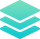Plagiarism Free Papers

All our papers are original and written from scratch. We will email you a plagiarism report alongside your completed paper once done.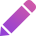Free Revisions

All papers are submitted ahead of time. We do this to allow you time to point out any area you would need revision on, and help you for free.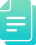Title-page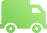Bibliography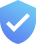Originality & Security

At Homework Valley, we take confidentiality seriously and all your personal information is stored safely and do not share it with third parties for any reasons whatsoever. Our work is original and we send plagiarism reports alongside every paper.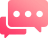Our agents are online 24/7. Feel free to contact us through email or talk to our live agents.

Try it now!

## Calculate the price of your order

We'll send you the first draft for approval by at
Total price:
\$0.00

How it works?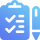Fill in the order form and provide all details of your assignment.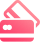Proceed with the payment

Choose the payment system that suits you most.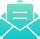Our Services

We work around the clock to see best customer experience.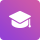## Flexible Pricing

Our prices are pocket friendly and you can do partial payments. When that is not enough, we have a free enquiry service.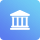When you need to elaborate something further to your writer, we provide that button.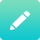Paper Submission

We take deadlines seriously and our papers are submitted ahead of time. We are happy to assist you in case of any adjustments needed.Customer Feedback

Your feedback, good or bad is of great concern to us and we take it very seriously. We are, therefore, constantly adjusting our policies to ensure best customer/writer experience.